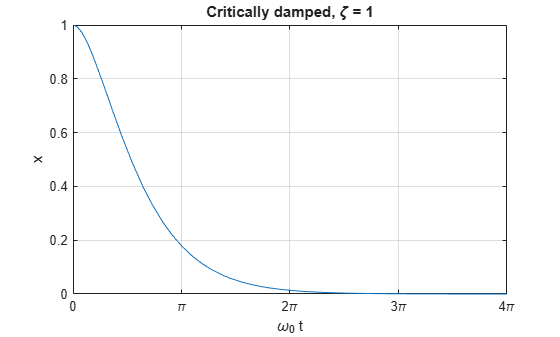Documentation

### This is machine translation

Mouseover text to see original. Click the button below to return to the English version of the page.

To view all translated materials including this page, select Country from the country navigator on the bottom of this page.

## The Physics of the Damped Harmonic Oscillator

This example explores the physics of the damped harmonic oscillator by

• solving the equations of motion in the case of no driving forces,

• investigating the cases of under-, over-, and critical-damping### Contents

1. Derive Equation of Motion

2. Solve the Equation of Motion (F = 0)

3. Underdamped Case ()

4. Overdamped Case ()

5. Critically Damped Case ()

6. Conclusion

### 1. Derive Equation of Motion

Consider a forced harmonic oscillator with damping shown below. Model the resistance force as proportional to the speed with which the oscillator moves.Define the equation of motion where

•is the mass

•is the damping coefficient

•is the spring constant

•is a driving force

syms x(t) m c k F(t) eq = m*diff(x,t,t) + c*diff(x,t) + k*x == F
eq(t) =Rewrite the equation usingand.

syms gamma omega_0 eq = subs(eq, [c k], [m*gamma, m*omega_0^2])
eq(t) =Divide out the mass. Now we have the equation in a convenient form to analyze.

eq = collect(eq, m)/m
eq(t) =### 2. Solve the Equation of Motion where F = 0

Solve the equation of motion using dsolve in the case of no external forces where. Use the initial conditions of unit displacement and zero velocity.

vel = diff(x,t); cond = [x(0) == 1, vel(0) == 0]; eq = subs(eq,F,0); sol = dsolve(eq, cond)
sol =Examine how to simplify the solution by expanding it.

sol = expand(sol)
sol =Notice that each term has a factor of, or, use collect to gather these terms

sol = collect(sol, exp(-gamma*t/2))
sol =The termappears in various parts of the solution. Rewrite it in a simpler form by introducing the damping ratio.

Substituting ζ into the term above gives:syms zeta; sol = subs(sol, ... sqrt(gamma^2 - 4*omega_0^2), ... 2*omega_0*sqrt(zeta^2-1))
sol =Further simplify the solution by substitutingin terms ofand,

sol = subs(sol, gamma, 2*zeta*omega_0)
sol =We have derived the general solution for the motion of the damped harmonic oscillator with no driving forces. Next, we'll explore three special cases of the damping ratiowhere the motion takes on simpler forms. These cases are called

• underdamped,

• overdamped, and

• critically damped.

### 3. Underdamped Case ()

If, thenis purely imaginary

solUnder = subs(sol, sqrt(zeta^2-1), 1i*sqrt(1-zeta^2))
solUnder =Notice the termsin the above equation and recall the identityRewrite the solution in terms of.

solUnder = coeffs(solUnder, zeta); solUnder = solUnder(1); c = exp(-omega_0 * zeta * t); solUnder = c * rewrite(solUnder / c, 'cos')
solUnder =solUnder(t, omega_0, zeta) = solUnder
solUnder(t, omega_0, zeta) =The system oscillates at a natural frequency ofand decays at an exponential rate of.

Plot the solution with fplot as a function ofand.

z = [0 1/4 1/2 3/4]; w = 1; T = 4*pi; lineStyle = {'-','--',':k','-.'}; fplot(@(t)solUnder(t, w, z(1)), [0 T], lineStyle{1}); hold on; for k = 2:numel(z) fplot(@(t)solUnder(t, w, z(k)), [0 T], lineStyle{k}); end hold off; grid on; xticks(T*linspace(0,1,5)); xticklabels({'0','\pi','2\pi','3\pi','4\pi'}); xlabel('t / \omega_0'); ylabel('amplitude'); lgd = legend('0','1/4','1/2','3/4'); title(lgd,'\zeta'); title('Underdamped');### 4. Overdamped Case ()

If, thenis purely real and the solution can be rewritten as

solOver = sol
solOver =solOver = coeffs(solOver, zeta); solOver = solOver(1)
solOver =Notice the termsand recall the identity.

Rewrite the expression in terms of.

c = exp(-omega_0*t*zeta); solOver = c*rewrite(solOver / c, 'cosh')
solOver =solOver(t, omega_0, zeta) = solOver
solOver(t, omega_0, zeta) =Plot the solution to see that it decays without oscillating.

z = 1 + [1/4 1/2 3/4 1]; w = 1; T = 4*pi; lineStyle = {'-','--',':k','-.'}; fplot(@(t)solOver(t, w, z(1)), [0 T], lineStyle{1}); hold on; for k = 2:numel(z) fplot(@(t)solOver(t, w, z(k)), [0 T], lineStyle{k}); end hold off; grid on; xticks(T*linspace(0,1,5)); xticklabels({'0','\pi','2\pi','3\pi','4\pi'}); xlabel('\omega_0 t'); ylabel('amplitude'); lgd = legend('1+1/4','1+1/2','1+3/4','2'); title(lgd,'\zeta'); title('Overdamped');### 5. Critically Damped Case ()

If, then the solution simplifies to

solCritical(t, omega_0) = limit(sol, zeta, 1)
solCritical(t, omega_0) =Plot the solution for the critically damped case.

w = 1; T = 4*pi; fplot(solCritical(t, w), [0 T]) xlabel '\omega_0 t'; ylabel 'x'; title 'Critically damped, \zeta = 1'; grid on; xticks(T*linspace(0,1,5)); xticklabels({'0','\pi','2\pi','3\pi','4\pi'});### 6. Conclusion

We have examined the different damping states for the harmonic oscillator by solving the ODEs which represents its motion using the damping ratio. Plot all three cases together to compare and contrast them.

zOver = pi; zUnder = 1/zOver; w = 1; T = 2*pi; lineStyle = {'-','--',':k'}; fplot(@(t)solOver(t, w, zOver), [0 T], lineStyle{1},'LineWidth',2); hold on; fplot(solCritical(t, w), [0 T], lineStyle{2},'LineWidth',2) fplot(@(t)solUnder(t, w, zUnder), [0 T], lineStyle{3},'LineWidth',2); hold off; textColor = lines(3); text(3*pi/2, 0.3 , 'over-damped' ,'Color',textColor(1,:)); text(pi*3/4, 0.05, 'critically-damped','Color',textColor(2,:)); text(pi/8 , -0.1, 'under-damped'); grid on; xlabel('\omega_0 t'); ylabel('amplitude'); xticks(T*linspace(0,1,5)); xticklabels({'0','\pi/2','\pi','3\pi/2','2\pi'}); yticks((1/exp(1))*[-1 0 1 2 exp(1)]); yticklabels({'-1/e','0','1/e','2/e','1'}); lgd = legend('\pi','1','1/\pi'); title(lgd,'\zeta'); title('Damped Harmonic Oscillator');#### Mathematical Modeling with Symbolic Math Toolbox

Get examples and videos